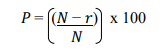# CAT Marks Vs Percentile: Past Year Trends, Section-wise Scores, and Calculation Process

Updated on 23rd June 2023 | 3:27 pm | #CATMarksVsPercentile

CAT is conducted once every year for admission into some of the top B-schools in the country.

CAT 2022 was held on 27 November 2022, in three different slots on the same day. The results of the exam were released on 21 December 2022.

Here are some key points to keep in mind regarding the CAT percentiles and marks:

• The scorecard provides the overall and sectional scaled scores and percentiles.
• Candidates must meet the individual percentile cutoffs of the B-schools to get calls.
• The percentiles are calculated based on the scaled scores.

Now let’s understand what marks and percentiles mean.

## What are Scaled Scores and Percentiles?

We are all familiar with the raw scores, the total marks calculated based on the correct and incorrect attempts of a candidate. As there are three slots in CAT with varying levels of difficulty per section, the raw scores of each candidate are converted to scaled scores to standardize the marks across all slots.

A percentile, on the other hand, is a reflection of how you have performed across test takers. For instance, the 90th percentile reflects that your test score is greater than 90% of the test takers. The higher the percentile the better. Both the CAT marks and percentile are different parameters that play a vital role in qualifying for the CAT exam.

The CAT scaled score is evaluated on the basis of CAT marks whereas the CAT Percentile is the total percentage of candidates who secured less than or equal to a specific candidate’s score.

This brings us to the question,

How much do you need to score in CAT to get a 99%ile?

## CAT 2022: Marks Vs Percentile

Here are the scores and total questions you needed in CAT 2022 for top percentiles to get calls from B-schools.

 Score Percentile Rank Net Qs 93.4 99.5 31 83.9 99 27 67.9 97 22 59.7 95 19 48.7 90 16 41.29 85 13 36.25 80 12 31.8 75 10 28 70 9 21.6 60 7 16.6 50 5

## Section-wise Marks and Percentiles

While the overall marks and percentile are important candidates must also keep in mind the sectional cutoffs. So, here are the number of questions to attempt accurately along with the scaled scores of all three sections of CAT to reach the 99%ile as per CAT 2022.

 Section Scaled Scores Number of Questions Verbal Ability & Reading Comprehension (VARC) 39.10 13 DILR 29.10 9 QA 33 11

### Marks Vs Percentile: VARC

There were a total of 24 questions in this section in CAT 2022 out of which 16 questions were from RC passages and the remaining 8 questions were from VA.

The VARC section comprises two sub-sections Reading Comprehension and Verbal Ability. The section focuses on grammar, vocabulary, para jumbles, para summary, odd sentence out, and para completion.

To get the 99th percentile in this section, you needed to get around 13 questions correct.

### Marks Vs Percentile: DILR

Owing to the complexity of the section and the fear it creates, the scores for this section are lower and thus relatively easier to achieve. 9 correct answers will help you enter the 99th percentile.

There are four sets in this section with 5 questions each. The sets are data-based reasoning sets and call for a knack for data and logical thinking. To do well in this section, you need a sound strategy and should steer clear of panic in case of a difficult DILR section.

### Marks Vs Percentile: QA

This section is focused on mathematics and requires aspirants to be well-versed in various mathematical formulae, equations, and shortcuts to do well. Candidates can focus on estimating the answer to the nearest answer in the MCQs to lessen the time spent per question.

To get into the 99 percentile in this section, you must aim for 11 correct attempts.

## Trends based on CAT 2022

Following the past year's trends, candidates can infer an estimated number of questions that they must answer correctly to get a good percentile. Here is an expected overview for CAT 2023-

 Sections Total Questions Per Section Number of Questions Verbal Ability & Reading Comprehension 24 13-15 Data Interpretation & Logical Reasoning 20 9-12 Quantitative Ability 22 11-13 Total no of Questions 66 27 - 33

## CAT 2022: Calculating Percentiles

The calculated percentile of each aspirant is given on the respective scorecard. The method of calculating the percentile is also provided by the convening body on the official website of CAT. Here is the method used to calculate the percentiles in CAT 2022:where,

P = Percentile of the candidate

N = Number of candidates who appeared for CAT 2022

r = The rank assigned to candidates based on their scaled scores

Note:

If the scaled score of two or more candidates is the same, they will be given the same rank (r). That is to say that if two candidates get the highest scaled score in VARC, they will be assigned rank 1. Also, the candidate to get the second highest scaled score will follow at rank 3.

The Final Percentile (P) will be rounded off to two decimal places.